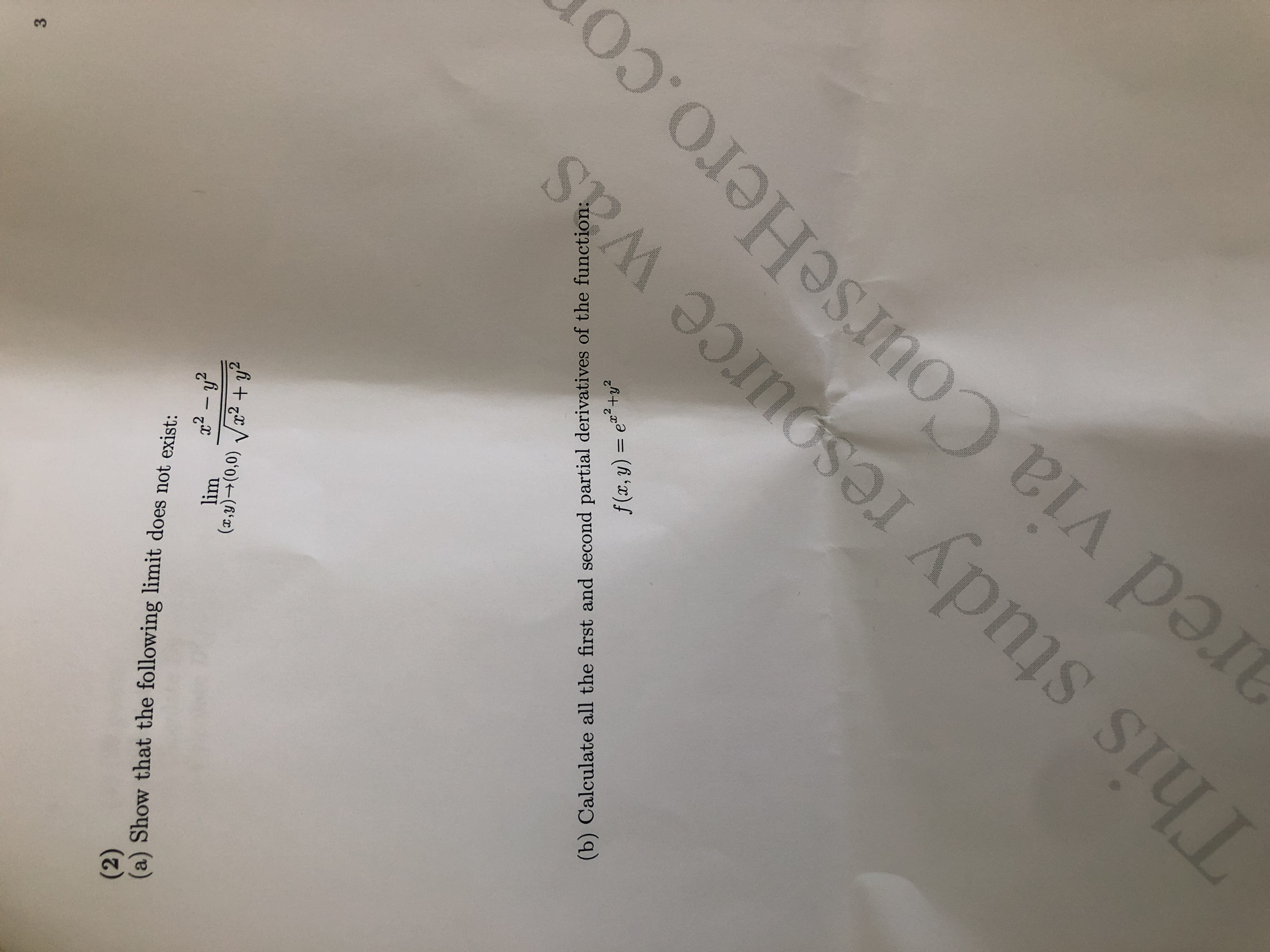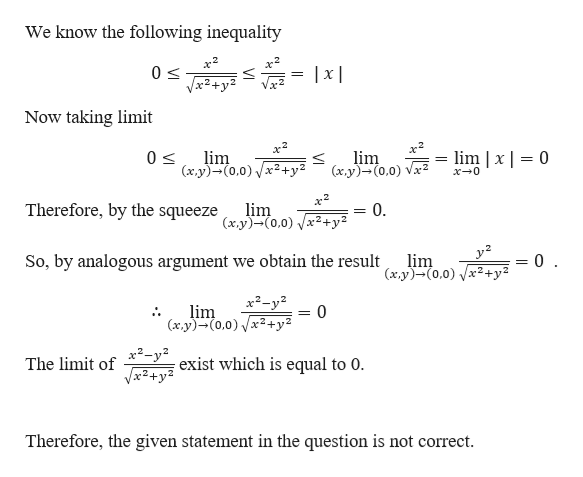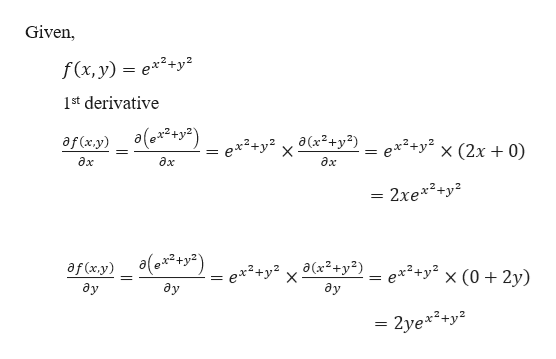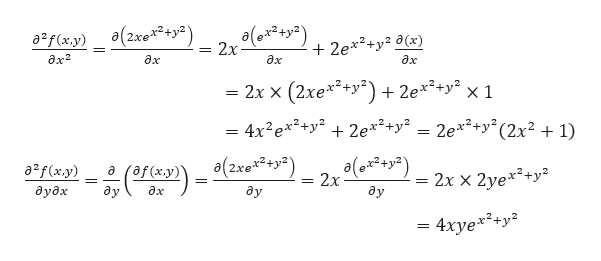# 3(2)(a) Show that the following limit does not exist:2y2lim(x,y)(0,0)2+y(b) Calculate all the first and second partial derivatives of the function:f (x, y) e+yThis study resource wasared via COurseHero.co

Question
37 views

Question 2 Praticehelp_outlineImage Transcriptionclose3 (2) (a) Show that the following limit does not exist: 2y2 lim (x,y)(0,0)2+y (b) Calculate all the first and second partial derivatives of the function: f (x, y) e+y This study resource was ared via COurseHero.co fullscreen
check_circle

Step 1

Part Ahelp_outlineImage TranscriptioncloseWe know the following inequality |x2+y2 Now taking limit x2 lim (axy)-(0.0) 2 lm x 0 lm 0 (xy)-(0,0) x0 Therefore, by the squeeze (x.y)(0.0) lim 0. y2 0 So, by analogous argument we obtain the result lim (x.y(0,0) x2-y lim (x.y)-(0,0)2+; 0 exist which is equal to 0. The limit of Therefore, the given statement in the question is not correct fullscreen
Step 2

Part b

Given,help_outlineImage TranscriptioncloseGiven, f(x, y) e*2+y2 1st derivative af (xy) ae _ex+y2 x a(x2+y?) . ex2+y2 x (2x 0) ax дх дх 2xe2ty2 = af (xy) (e+y2) ay ex2+y2 x a(x2+y?) ay ex2+y? x (0 + 2y) ay = 2ye*2+y fullscreen
Step 3

2nd...help_outlineImage Transcriptionclose2f(xy)(2xe2+y) 2e*2+y2 x) 2x ax2 дх дх дх = 2x X (2xe*+y)2e*ty' x 1 4x2 e2+y 2e*+y = 2e**+y"(2x2 1) a2f(x.y) af (xy) a 2x x 2ye*2+y 2x дудх ay ay дх ay = 4xye*+y* fullscreen

### Want to see the full answer?

See Solution

#### Want to see this answer and more?

Solutions are written by subject experts who are available 24/7. Questions are typically answered within 1 hour.*

See Solution
*Response times may vary by subject and question.
Tagged in

### Limits